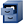# Unit Risk Estimate (URE)Note: EPA no longer updates this information, but it may be useful as a reference or resource.

The URE is the upper-bound excess lifetime cancer risk estimated to result from continuous exposure to an agent at a concentration of 1 µg/m3 in air. The interpretation of the URE would be as follows: if the URE = 1.5 x 10-6 µg/m3, 1.5 excess tumors are expected to develop per 1,000,000 people if exposed daily for a lifetime to 1 µg of the chemical in 1 cubic meter of air. UREs are considered upper bound estimates, meaning they represent a plausible upper limit to the true value. (Note that this is usually not a true statistical confidence limit.) The true risk is likely to be less, but could be greater.

#### Reference Concentration (RfC)

The RfC is an estimate (with uncertainty spanning perhaps an order of magnitude) of a continuous inhalation exposure to the human population (including sensitive subgroups) that is likely to be without an appreciable risk of deleterious effects during a lifetime. It can be derived from various types of human or animal data, with uncertainty factors generally applied to reflect limitations of the data used.

#### Upper Confidence Limit (UCL)

The UCL is the upper bound of a confidence interval around any calculated statistic, most typicially an average. For example, the 95% confidence interval for an average is the range of values that will contain the true average (i.e., the average of the full statistical population of all possible data) 95% of the time. In other words, 97.5% of UCLs calculated with different data sets from the same statistical population will exceed the true average of that population. EPA has based most UREs on the UCL of response data or of fitted curves, to avoid underestimating the true URE in the face of uncertainty.

#### Maximum Likelihood Estimate (MLE)

The most accurate MLE is, by definition, the mode of a data set (i.e., the most frequent observation), although when data are too limited to identify a clear mode the average or the median of the data are usually substituted. For some HAPs for which adequate human data exist, EPA has based the URE on the MLE for response data or for fitted curves.